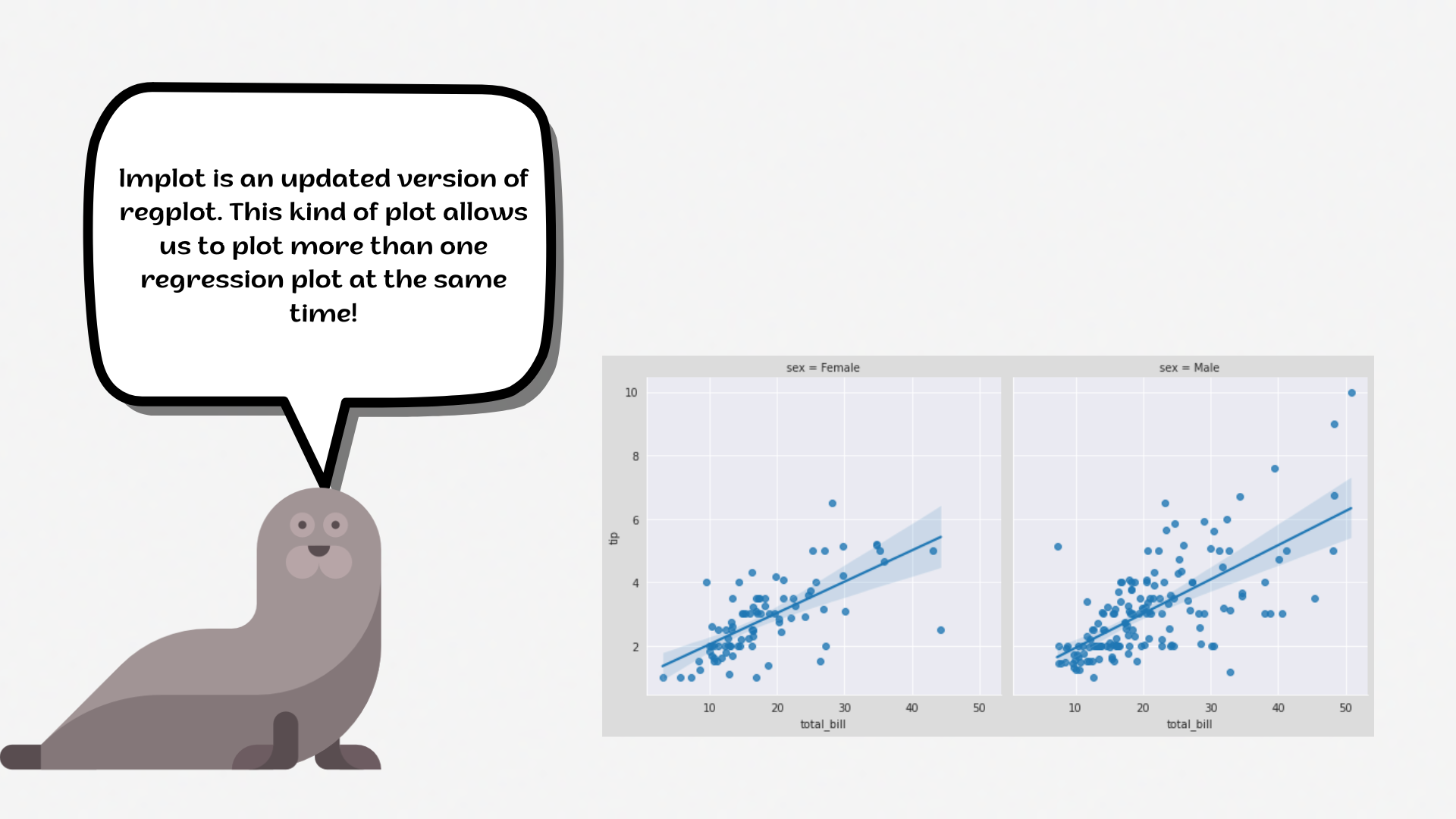Course Content

# Deep Dive into the seaborn Visualization

1. Light Start

2. Distributions of Data

3. Categorical Plot Types

4. Matrix Plots

5. Multi-Plot Grids

6. Regression Models

Deep Dive into the seaborn Visualization

##LmplotNote

`lmplot` = `FacetGrid` + `regplot`1. Set the `'darkgrid'` style with the `'lightpink'` `figure.facecolor`.
2. Create a `lmplot` using the `seaborn` library:
• Set the `x` parameter equals the `'total_bill'`;
• Set the `y` parameter equals the `'tip'`;
• Set the `hue` parameter equals `'smoker'`;
• Set the `col` parameter equals the `'sex'`;
• Set the `'o'` and `'x'` `markers`;
• Disable the `legend_out` parameter;
• Set the `'crest'` `palette`;
• Set the data;
• Display the plot.Everything was clear?

Section 6. Chapter 2